Home    |    Teacher    |    Parents    |    Glossary    |    About UsEmail this page to a friendResources· Cool Tools · Formulas & Tables · References · Test Preparation · Study Tips · Wonders of MathSearchTransforms (Math | Advanced | Transforms)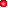Laplace Transformsf(x) =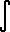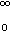e^(-xt) g(t) dt (Laplace Transform)f(x) =e^(-xt) g(t) d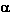(t) (Laplace-Stieltjes Transform)f2(x) = L{L{g(t)}} =g(t)/(x+t) dt (Stieltjes Transform)Fourier Transformsf(x) = 1/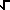(2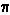)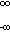g(t) e^(i tx) dt (Fourier Transform)f(x) =(2/)g(x) cos(xt) dt (Cosine Transform)f(x) =(2/)g(x) sin(xt) dt (Sine Transform)Power Series Transformf(x) =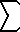(k=0..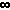) g(k) x^k